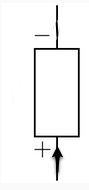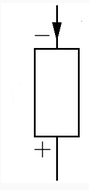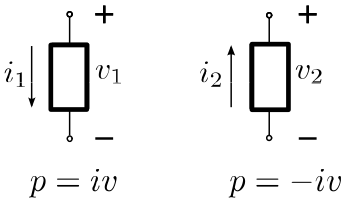Formulae, constants, laws, etc.

• Coulomb = C
• Electrons per coulomb: $$6.241509745 * 10^{18}$$
• Coulombs per electron: $$1.602 x 10^{-19}$$
• Coulomb’s Law:
 $\left F \right = k_{e}\frac{q_{1}q_{2}}{r^{2}}$
• … where
• $$k_{e}$$ is a constant
• $$q_{1}$$ is the charge of the first particle in Coulombs
• $$q_{2}$$ is the charge of the second particle in Coulombs
• r is the distance between them in meters
• F is the force in newtons
• m is meters
• N is Newtons
• $$k_{e}=8.988 \ast 10^{9} \frac{Nm^2}{C^2}$$
• Meter: Abbreviated m.
• Centimeter: Abbreviated cm.
• Newton: Unit of force. Force needed to accelerate 1 kilogram of mass at the rate of 1 meter per second squared. Abbreviated N.
• Amp: Unit of current. Abbreviated A.
• Amps = Coulombs / seconds
• $$A=\frac{C}{s}$$
• Joule: Unit of work (or energy expended). Abbreviated J. Newtons * meters.
• 1 Joule = passing an electric current of one amp through a resistance of one ohm for one second
• Other measures of work/energy expended:
• Watts * seconds
• Coulombs * volts
• Volts are joules divided by coulombs. i.e. 1V = $$\frac{1J}{1C}$$
• Watts:
• Joules per second
• Volts * Amps
• Current arrows
##### Forward (positive):From plus, through to minus is forward (consuming power).

##### Reverse (negative):From minus, through to plus is backward (generating power).

For voltages, + connected to + is forward.

##### Another way of diagramming the same thing• Ohm’s Law:
• v = iR
• If current arrow is from minus, through to plus, then use v = -iR
• $$\Omega=\frac{V}{A}$$
• Volts / Amps
• Metric prefixes
• k = kilo = $$10^{3}$$
• M = mega = $$10^{6}$$
• c = centi = $$10^{-2}$$
• m = milli = $$10^{-3}$$
• $$\mu$$ = micro = $$10^{-6}$$
• Kirchhoff’s Current Law: The sum of all currents entering a node = the sum of all currents leaving a node.
• Resistors in series: $$R_{1} + R_{2} + R_{3}$$
• Resistors in parallel: $$\left ( \frac{1}{R_{1}} + \frac{1}{R_{2}} + \frac{1}{R_{3}} \right )^{-1}$$ or use $$\frac{R_1 R_2}{R_1 + R_2}$$

• Voltage divider (using resistors in series): $$v_1=\frac{R_1}{R_1+R_2}v$$

• Current divider (using resistors in parallel): $$i_1=\frac{R_2}{R_1+R_2}i$$

• Capacitance in Farads: $C = \frac{Q}{V}$
• 1 microfarad = 1 $$\mu$$F $$= 1 \times 10^{-6}$$ farad
• 1 picofarad = 1 pF = $$1 \times 10^{-12}$$ farad = 1 micro microfarad